# Create a functional basis process¶

The objective of this example is to define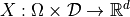a multivariate stochastic process of dimension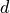where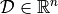, as a linear combination of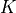deterministic functions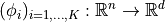: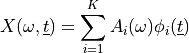where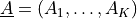is a random vector of dimension.

We suppose that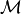is discretized on the meshwhich has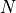vertices.

A realization of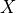onconsists in generating a realization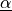of the random vector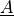and in evaluating the functions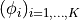on the mesh.

If we note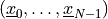the realization of, where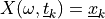, we have: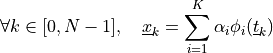import openturns as ot
import openturns.viewer as viewer

ot.Log.Show(ot.Log.NONE)


Define the coefficients distribution

mu = [2.0] * 2
sigma = [5.0] * 2
R = ot.CorrelationMatrix(2)
coefDist = ot.Normal(mu, sigma, R)


Create a basis of functions

phi_1 = ot.SymbolicFunction(["t"], ["sin(t)"])
phi_2 = ot.SymbolicFunction(["t"], ["cos(t)^2"])
myBasis = ot.Basis([phi_1, phi_2])


Create the mesh

myMesh = ot.RegularGrid(0.0, 0.1, 100)


Create the process

process = ot.FunctionalBasisProcess(coefDist, myBasis, myMesh)


Draw a sample

N = 6
sample = process.getSample(N)
graph = sample.drawMarginal(0)
graph.setTitle(str(N) + " realizations of functional basis process")
view = viewer.View(graph)